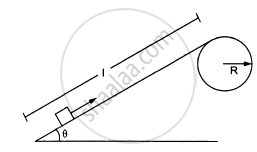Advertisement Remove all ads

# Figure ( Following ) Shows a Smooth Track Which Consists of a Straight Inclined Part of Length L Joining Smoothly with Circular Part. .Assuming that Projection-speed is Only Slightly Greater than ν 0 - Physics

Numerical

Figure ( following ) shows a smooth track which consists of a straight inclined part of length l joining smoothly with the circular part. A particle of mass m is projected up the incline from its bottom.Assuming that the projection-speed is only slightly greater than $\nu_0$ , where will the block lose contact with the track?Advertisement Remove all ads

#### Solution

Let the loose contact after making an angle θ.

$\frac{\text{m} \nu^2}{R} = \text{mg} \cos \theta$

$\Rightarrow \nu^2 = \text{Rg} \cos \theta . . . (\text{i})$

$\text{Again,} \frac{1}{2}\text{m}\nu^2 = \text{mg } \left( R - R \cos \theta \right)$

$\Rightarrow \nu^2 = 2gR \left( 1 - \cos \theta \right) . . . (\text{ii})$

$\text{From (i) and (ii), }= \cos^{- 1} \left( \frac{2}{3} \right)$
$\Rightarrow \theta = \cos^{- 1} \left( \frac{2}{3} \right)$

Is there an error in this question or solution?
Advertisement Remove all ads

#### APPEARS IN

HC Verma Class 11, 12 Concepts of Physics 1
Chapter 8 Work and Energy
Q 62.3 | Page 137
Advertisement Remove all ads
Advertisement Remove all ads
Share
Notifications

View all notifications

Forgot password?